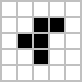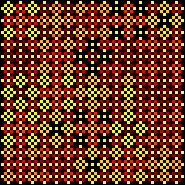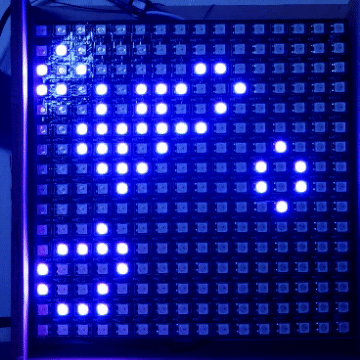# Task #12: Signs of new life

As part of doing the Sunrise task, @zranger1 built a object focused framework, so his planets/etc would move around the sun. I still need/want to turn that into a more generic solution, enabling “boids” and other independent behavior models to be built.

There is another sort of basic framework when it comes to Matrixes: it uses an array, for each pixel, to pay attention to nearby pixels, and thus do other sorts of behavior models. The difference is that the first is focused on the object(s), while this one is focused on the matrix of positions.

One classic example is Life by John Conway.
Given a field of pixels, run thru each pixel and look at the surrounding pixels:

1.Any lit pixel with fewer than two live neighbors dies (turns off), as if by loneliness. (Neighbors are the 8 surrounding pixels, and you can either consider the edges as off, or wrap around)

1. Any lit pixel with more than three lit neighbors dies (turns off), as if by overcrowding.

2. Any unlit pixel with exactly three lit neighbors lights up.

put another way, for clarity (and logic)

1. Any lit pixel with two or three lit neighbours stay lit.
2. Any unlit pixel with three lit neighbours becomes a lit pixel.
3. All other lit pixels turn off in the next generation. Similarly, all other unlit pixels stay off.

I was surprised I didn’t see a Life implementation
in the pattern collection. It’s time to fix that, and this is the perfect bit of coding where there are dozens of ways to do it, and lots of potential shortcuts so golfing will be interesting too.

Adding color is optional (I don’t recommend using white, just for power reasons), but you could potentially make it color sensitive, like blue pixels count as 2, or green pixels always stay alive, etc… (See below for more ideas)

Usually a random starting layout is best… Or you can use the classic layout:which is known to run for quite a while. (the dark cells n that images are the LIT ones, to be clear)

Beyond Life, this sort of code can be used for things like sand sliding around, water sloshing and more… Basically it’s a good core way to simulate.

There are lots of implementations out there, if you need some help… And of course, many of us will also be happy to help if you get stuck.

It’s been a long time since I played with Life myself. So I dug into some of the existing programs, and found some amazing resources…

And while cellular automata (which all of this is) does have a 1D pattern in the PB pattern archive… its time for some 2D ones… cause with some additional/different rules and adding colors, you can make amazing patterns like this:

Or thisAnd so many others:

http://www.mirekw.com/ca/ca_gallery.html

1 Like

I’ve got something in mind, but will be late to the party for this (and for making art as well). To my surprise, I’m travelling this week. First time in quite a while. Air travel hasn’t changed. Fewer people, but even less redundancy in the system, which means it behaves pretty much the way it always has…

1 Like

I took a crack at this today.

I was re-reading Wolfram’s book and found “Continuous cellular automata”, which map values from 0 to 1, and thought - hey, that sounds a lot like Pixelblaze’s superpower!

Code is up on the library. You can get some really diverse stuff out of CA, and for the uninitiated, its sensitivity to initial conditions is always amazing.

3 Likes

based on the particular page # comments (mentioned in @jeff’s code itself) about his inspiration, I was delighted to see Wolfram’s book “A New Kind of Science” is online and easily browsable:

2 Likes
2 Likes

and one more, cause this rabbit hole has so many neat places:

First (but found this digging for the next thing): Wolfram Cellular Automata used to generate music

This takes CA and makes it into music.

It take music and modifies the CA to react to it.
And… the website link was dead. Wayback Archive yielded just 1 result (thankfully!)

a second video:

and CODE:

So @jeff you (or someone else) can add input via the sound board!
and then the patterns would change based on what the PB hears.

Ok… just to demonstrate that I can sometimes do an assignment as specified, and because every platform should have one, here’s a basic implementation of Conway’s life. It’s initialized randomly, with UI to control the pattern lifetime and framerate. (I haven’t given up on overkill – the cyclical CA engine is coming as soon as I get it cleaned up and commented. There are just endless cool things to do with cellular automata…)``````/* Conway's Life 2D

Straightforward implementation of Conway's Life for 2D matrices.
https://en.wikipedia.org/wiki/Conway%27s_life

Requires a 2D display and appropriate mapping function.

Version  Author        Date
1.0.0    JEM(ZRanger1) 05/02/2021
*/

// display size - enter the dimensions of your matrix here
var width = 16;
var height = 16;

// Global variables for rendering
var buffer1 = array(height);   // main drawing surface
var buffer2 = array(height);   // secondary drawing surface
var pb1, pb2;                  // buffer pointers for swapping

// Variables for animation control
export var speed = 100;        // milliseconds per frame
export var lifetime = 9000;    // how long (in milliseconds) do we let each "pattern" run
var frameTimer = 9999;         // accumulator for simulation frame timer
var patternTimer = 9999;       // accumulator for pattern life timer.
var t1;                        // color modulation timer

// UI
export function sliderSpeed(v) {
v = 1-v;
speed = 1000 * v * v;
}

// most Life patterns are not very long lived, so to keep the display
// interesting, we'll have to restart periodically. This slider controls
// how long the pattern runs before reinitializing, from 1 to 30 seconds.
lifetime = 1000 + (v * 29000)
}

// initialize by randomly seeding a specified percentage of cells
function seedCA(prob) {
for (var y = 1; y < height; y++) {
for (var x = 1; x < width; x++) {
pb2[x][y] = (random(1) < prob);
}
}
}

// create 2 x 2D buffers for calculation
function allocateFrameBuffers() {
for (var i = 0; i < height; i ++) {
buffer1[i] = array(width);
buffer2[i] = array(width);
}
pb1 = buffer1;
pb2 = buffer2;
}

function swapBuffers()  {
var tmp = pb1; pb1 = pb2; pb2 = tmp;
}

function sumNeighborhood8(x,y,buffer) {
// precalculate wrapped neighbor indices.
xm = (x > 0) ? x - 1 : width - 1;
ym = (y > 0) ? y - 1 : height - 1;
xp = (x + 1) % width;
yp = (y + 1) % height;

// return number of living neighbor cells
return buffer[x][ym] + buffer[x][yp] + buffer[xm][y] + buffer[xp][y] +
buffer[xm][ym] + buffer[xp][ym] + buffer[xm][yp] + buffer[xp][yp];
}

function doGeneration() {
swapBuffers();

// implement the rules of Life:  If a cell has fewer than two living neighbors,
// it dies of lonliness, if more than three, it dies of overcrowding.
// if an empty cell has exactly three neighbords, it spawns new life
// otherwise, with 2 or 3 neighbors, the cell lives on to the next generation
for (var y = 1; y < height; y++) {
for (var x = 1; x < width; x++) {
var sum = sumNeighborhood8(x,y,pb1);
if (sum < 2 || sum > 3) { pb2[x][y] = 0; }
else if (sum == 3) { pb2[x][y] = 1; }
else { pb2[x][y] = pb1[x][y]; }
}
}
}

// Initialization
allocateFrameBuffers();

export function beforeRender(delta) {
frameTimer += delta;
patternTimer += delta;

// timer to give us a little color change
t1 = time(0.08);

// if we've reached the end of a pattern's allotted lifespan
// start a new one
seedCA(0.3);
patternTimer = 0;
}

// if it's time for a new frame, calculate the next generation
if (frameTimer > speed) {
doGeneration();
frameTimer = 0;
}
}

export function render2D(index, x, y) {
// convert x and y to array indices
x = floor(x * width);
y = floor(y * height);
hsv(t1,1,(pb2[x][y] > 0) * 0.6);
}
``````
4 Likes

Just uploaded Cyclic Cellular Automata2D to the library. Source below, and video when I get a sec to edit and upload it. Take my word for it though, this one makes very pretty pictures. I think I’m going to consider it my Task #11 (Make Art!) entry too!

It has two modes, selected by slider

• mode 0, a Greenberg-Hastings CCA, which models excitable media, and produces swirling, painterly patterns, and
• mode 1 - a “normal” cyclic CA, which is simpler and more liquid.

If you enable the “advanced UI” by decommenting the block of sliders, you can mess with a great many parameters and produce some really interesting things. But like Jeff’s continuous CA, (which… Wow, I love that!!) it is very sensitive to initial conditions, so a little patience is required. Tweak a parameter, and sit back and watch it evolve for a while.

Enough talk! On to the blinky lights!

``````/* Cyclic Cellular Automata 2D

Displays a cyclic cellular automaton, and a variant of the Greenberg-Hastings CCA:

https://en.wikipedia.org/wiki/Greenberg%E2%80%93Hastings_cellular_automaton

This flavor of CA is used to model "excitable" systems -- a system that can
activate, and support the passage of a wave of some sort, after which it
must "rest" for some period of time before another wave can pass.

A forest fire is the canonical example of this kind of system...

Requires a 2D LED array and appropriate pixel mapper.

UI Sliders:
Speed:      Controls number of milliseconds per frame
Lifetime:   How long a given pattern runs before being re-randomized
A lifetime of 0 means "forever"
Threshold:  Number of correctly valued neighbors required to advance to
the next state.
States:     Number of allowed states for each cell.
Excited:    Percentage of cells to initialize to the excited state
Refractory: Percentage of cells to initialize to a random refractory level
Mode:       Switches between Greenberg-Hastings and "normal" cyclic CA.  Flipping
the mode switch restores default values for the more sensitive
parameters.

Cells are randomly initialized according to the current mode and parameter set.
Some initial condition sets may "fizzle" and die out.  If this occurs, the
pattern will automatically re-initialize.

The default settings produce mostly "good" results, but this pattern rewards
experimentation and a bit of patient watching.  It can produce beautiful visuals
that would be near impossible to make any other way!

Version  Author        Date
1.0.0    JEM(ZRanger1) 05/03/2021
*/

// display size - enter the dimensions of your matrix here
var width = 16;
var height = 16;

// Global variables for rendering.
var buffer1 = array(height);   // main drawing surface
var buffer2 = array(height);   // secondary drawing surface
var pb1, pb2;                  // buffer pointers for swapping
export var numStates = 24;
export var speed = 60;         // milliseconds per frame
export var lifetime = 10000;   // how long between reinitializations
export var excited = 0.03;     // % cells initialized to "excited" state
export var refractory = 0.64;  // % cells initialized to random refractory level
export var threshold = 1;      // minimum activation level
var mode = 0;                  // 0 = Greenberg-Hastings, 1 = Cyclic CA
var calcNextGen = doGenerationGH;
var nextVal = 1;
var frameTimer = 9999;         // accumulator for simulation timer
var patternTimer = 9999;       // accumulator for pattern lifetime

// UI
export function sliderSpeed(v) {
speed = 1000 * v * v;
}

// lifetime of pattern in milliseconds.  0 == forever
}

// Set operating mode: 0 == GBH, 1 = CCA
export function sliderMode(v) {
mode = (v > 0.5);

calcNextGen = mode ? doGenerationCCA : doGenerationGH;

if (mode == 0) {
threshold = 1;
numStates = 24;
excited = 0.03;
refractory = 0.64;
}
else {
threshold = 3;
numStates = 3;
}
}

// Uncomment the block below if you want to play with more parameters.
// Enabling these sliders breaks the Mode slider's automatic setting of
// reasonable defaults for the more sensitive parameters, so if you like
// your settings, take note of them before you do this.  And remember
//
// "With great power comes great responsibility" - Spider-man
/*
export function sliderThreshold(v) {
threshold = 1+floor(v * 3);
}

export function sliderStates(v) {
numStates = floor(v * 32);
}

// allows a maximum of 20% of cells to be initially excited
export function sliderExcited(v) {
excited = 0.20 * v * v;
}

// allows a maximum of 80% of cells to be initialied to the
// refractory state
export function sliderRefractory(v) {
refractory = 0.8 * v * v;
}
*/

// Master CA Initializer
// init the array to a random(ish) state appropriate for the
// current mode.
function seedCA() {
if (mode) {
seedCCA()
} else {
seedGH(excited,refractory);
}
}

// init classic CCA
// Set all cells to random activation level
function seedCCA() {
var x,y,i;

lastState = buffer1;
currentState = buffer2;

for (y = 0; y < height; y++) {
for (x = 0; x < width; x++) {
currentState[x][y] = floor(random(numStates));
lastState[x][y] = currentState[x][y];
}
}
}

// Init Greenberg-Hastings CA
// Set cells given probability of excited and refactory levels.
// TODO - rework this to shuffle instead of generating random coord pairs.
function seedGH(probX,probR) {
var x,y,i;

pb1 = buffer1;
pb2 = buffer2;

// zero arrays
for (y = 0; y < height; y++) {
for (x = 0; x < width; x++) {
pb1[x][y] = 0;
pb2[x][y] = 0;
}
}

// distribute excited cells
probX = floor(pixelCount * probX)
for (i = 0; i < probX;) {
x = random(width); y = random(height);
if (pb2[x][y] == 0) {
pb2[x][y] = 1
i++;
}
}

// distribute refactory cells
probR = floor(pixelCount * probR)
for (i = 0; i < probR;) {
x = random(width); y = random(height)
if (pb2[x][y] == 0) {
pb2[x][y] =  2+floor(random(numStates - 2))
i++;
}
}
}

// create 2 x 2D buffers for calculation
function allocateFrameBuffers() {
for (var i = 0; i < height; i ++) {
buffer1[i] = array(width);
buffer2[i] = array(width);
}
pb1 = buffer1;
pb2 = buffer2;
}

function swapBuffers()  {
var tmp = pb1; pb1 = pb2; pb2 = tmp;
}

// counts excited neighbors
function sumNeighborhood4(x,y,buffer) {
return (buffer[x][ym] == nextVal) + (buffer[x][yp] == nextVal) +
(buffer[xm][y] == nextVal) + (buffer[xp][y] == nextVal);
}

function sumNeighborhood8(x,y,buffer) {
return (buffer[x][ym] == nextVal) + (buffer[x][yp] == nextVal) + (buffer[xm][y] == nextVal) +
(buffer[xp][y] == nextVal) + (buffer[xm][ym] == nextVal) + (buffer[xp][ym] == nextVal) +
(buffer[xm][yp] == nextVal) + (buffer[xp][yp] == nextVal);
}

var xm,xp,ym,yp;
function doGenerationGH() {
swapBuffers();
nextVal = 1;

for (var y = 0; y < height; y++) {
yp = (y + 1) % height;
ym = (y > 0) ? y - 1 : height - 1;

for (var x = 0; x < width; x++) {
xm = (x > 0) ? x - 1: width - 1;
xp = (x + 1) % width;

if (pb1[x][y] == 0) {
pb2[x][y] = (sumNeighborhood4(x,y,pb1) >= threshold);
}
else {
pb2[x][y] = (pb1[x][y] + 1) % numStates;
}
sum += pb2[x][y];
}
}
}

function doGenerationCCA() {
swapBuffers();

for (var y = 0; y < height; y++) {
yp = (y + 1) % height;
ym = (y > 0) ? y - 1 : height - 1;

for (var x = 0; x < width; x++) {
xm = (x > 0) ? x - 1: width - 1;
xp = (x + 1) % width;

nextVal = (pb1[x][y] + 1) % numStates;
var s = sumNeighborhood8(x,y,pb1);
pb2[x][y] = (s >= threshold) ? nextVal : pb1[x][y];
sum += (pb2[x][y] != pb1[x][y])
}
}
}

// Initialization
allocateFrameBuffers();

export function beforeRender(delta) {
frameTimer += delta;
patternTimer += delta;

// if the pattern hasn't died, and it's time for a new pattern,
// reinitialize the array.
seedCA(excited,refractory);
patternTimer = 0;
}

if (frameTimer > speed) {
sum = 0;
calcNextGen();
frameTimer = 0;
}
}

// The "sum" variable simply lets us know if any pixels have changed since the last
// frame.  If not, the CA has died, and we need to start a new one, which we'll
// do the next time beforeRender() is called.
var sum = 0;
export function render2D(index, x, y) {
x = (x * width);
y = (y * height);
var cell = pb2[x][y];
var state = cell / numStates;
hsv(state,1, wave(state));
}
``````
2 Likes

YESSS!!! Wow this one is amazing, Jon. Nice!!

1 Like

Here’s some video. It runs for about 30 seconds in Greenberg-Hastings mode (mode 0), then switches to “normal” CCA (mode 1), then back to GH again at the end.

(I’m still not thrilled with my 2D pattern videos, but I’m learning every time I make one. People filming strips tend to let them blow out and saturate a little because it looks good - gives that glowing plasma effect. On a 2D matrix, keeping the natural vibrancy of the LEDs is a trickier balance. )

2 Likes

One more for the rabbit hole:

1 Like

One more for the rabbit hole:

And for the sake of completion:
See Neat AI does Lenia - Conway's game of life arrives in the 21st century - YouTube
At 4 minutes in, has a Life simulating Life excerpt.

I really like the patterns this code generates however it doesn’t translate well to an 8X8 matrix.
Can anyone suggest a small matrix code on this site?

Sadly it won’t translate well to something really small. You could simulate a larger field and then scale it down but honestly, it’ll lose a lot of the details and just make mushy shapes, if anything.

Fantastic generated pattern…I always live what you come up with.